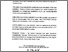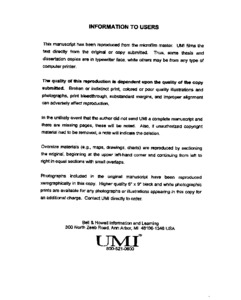Title:

# A unified study of bounds and asymptotic estimates for renewal equations and compound distributions with applications to insurance risk analysis

Cai, Jun (1998) A unified study of bounds and asymptotic estimates for renewal equations and compound distributions with applications to insurance risk analysis. PhD thesis, Concordia University.Preview Text (application/pdf) NQ39619.pdf 4MB

## Abstract

This thesis consists of a unified study of bounds and asymptotic estimates for renewal equations and compound distributions and gives applications to aggregate claim distributions, stop-loss premium and ruin probabilities with general claim sizes and especially with heavy-tailed distributions. Chapter 1 presents the probability models of compound distributions and renewal equations in insurance risk analysis and gives the summary of the results of this thesis. In Chapter 2, we develop a general method to construct analytical bounds for solutions of renewal equations. Two-sided exponential and linear estimates for the solutions are derived by this method. A generalized Cramér-Lundberg condition is proposed and used to obtain bounds and asymptotic formulae with NWU distributions for the solutions. Chapter 3 discusses tails of a class of compound distributions introduced by Willmot (1994) and gives uniformly sharper bounds, both with the results obtained in Chapter 2 and renewal theory. The technique of stochastic ordering is employed to get simplified bounds for the tails and to correct the errors of the proofs of some previous results. In Chapter 4, we derive two-sided estimates for tails of a class of aggregate claim distributions, and especially give upper and lower bounds for compound negative binomial distributions both with adjustment coefficients and with heavy-tailed distributions. For the latter case, Dickson's (1994) condition plays the same role as the Cramér-Lundberg condition. Chapter 5 is devoted to the aging property of compound geometric distributions and its applications to stop-loss premiums and ruin probabilities. By the aging property, general upper and lower bounds for the stop-loss premium of the class of compound distributions discussed in Chapter 3 are derived, which apply to any claim size distribution. Also, two-sided estimates for the stop-loss premium of negative binomial sums are obtained both under the Cramér-Lundberg condition and under Dickson's condition. General upper and lower bounds for ruin probabilities are also considered in this chapter. Chapter 6 gives a detailed discussion of the asymptotic estimates of tails of convolutions of compound geometric distributions. Asymptotic estimates for these tails are given under light, medium and heavy-tailed distributions, respectively. Applications of these results are given to the ruin probability in the diffusion risk model. Also, two-sided bounds for the ruin probability are derived by a generalized Dickson condition, which applies to any positive claim size distribution. Finally, we give some examples and consider numerical comparisons of bounds with asymptotic estimates.

Divisions: Concordia University > Faculty of Arts and Science > Mathematics and Statistics Thesis (PhD) Cai, Jun x, 130 leaves ; 29 cm. Concordia University Ph.D. Mathematics 1998 Garrido, Jose QA 273.6 C35 1998 522 Concordia University Library 27 Aug 2009 17:12 13 Jul 2020 19:46 https://concordiauniversity.on.worldcat....
All items in Spectrum are protected by copyright, with all rights reserved. The use of items is governed by Spectrum's terms of access.

Repository Staff Only: item control pageResearch related to the current document (at the CORE website)
Back to top